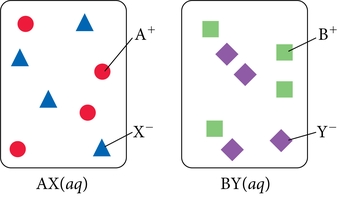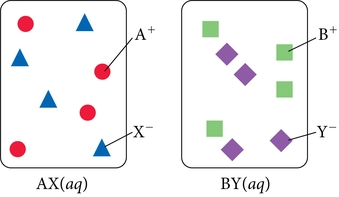# Problem: Consider the generic ionic compounds with the formulas AX and BY and the following solubility rules:AX - soluble; BY - soluble; AY - soluble; BX - insolubleLet circles represent A+ ions; squares represent B+ ions; triangles represent X–; and diamonds represent Y– ions. We represent solutions of the two compounds (AX and BY) as follows:Draw the correct molecular-level representation showing the solid that precipitates out of the solution as a result of mixing the two solutions.

###### FREE Expert SolutionAX(aq) + BY(aq) →

double displacement reaction

AX(aq) + BY(aq) → AY(aq) + BX(s)

BX - insoluble → precipiate

93% (95 ratings)###### Problem Details

Consider the generic ionic compounds with the formulas AX and BY and the following solubility rules:

AX - soluble; BY - soluble; AY - soluble; BX - insoluble

Let circles represent A+ ions; squares represent B+ ions; triangles represent X; and diamonds represent Y ions. We represent solutions of the two compounds (AX and BY) as follows:Draw the correct molecular-level representation showing the solid that precipitates out of the solution as a result of mixing the two solutions.

Frequently Asked Questions

What scientific concept do you need to know in order to solve this problem?

Our tutors have indicated that to solve this problem you will need to apply the Molecular Equations concept. You can view video lessons to learn Molecular Equations. Or if you need more Molecular Equations practice, you can also practice Molecular Equations practice problems.